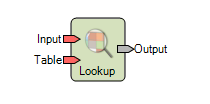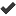# Lookup

## ERDAS IMAGINE Help

HGD_Variant
16.6
HGD_Product
ERDAS IMAGINE
HGD_Portfolio_Suite
Producer

Category: Analysis

Function Type: Point (see Operator Types in Spatial Modeler)• Description

Lookup maps input values through at lookup table using bin functions.

If Table has an associated bin function, the values in Input will be converted to bin numbers, then the bin number will be used as an index into the lookup table.

If Table does not have an associated bin function, Input is converted to integer, and this number is used as an index to the lookup table.

If Input is binary, integer, float, or complex, the output is the same data type as Table.

Supported Data Types

Input

Output

Binary

Binary

Integer

Integer

Float

Float

Complex

Complex

Color

Color

String

String

If Input is color and Table is float or color, Output is color. If Input is color and Table is any other type, Output is undefined type. String type for the input is not supported.

Input may be any object type. Table is a Table. Output is the same object type and size as Input.

Exception: If Input is single layer Raster and Table is color, Output is a three layer Raster. If Input is Raster and Table is color, the number of layers in Input must be one or three.

• Connections

Name

Objects Supported

Required

Input

Scalar, Matrix, Table, RasterTable

Scalar, Matrix, Table, RasterOutput

Scalar, Matrix, Table, Raster

Syntax

Lookup ( <Input>, <Table> )